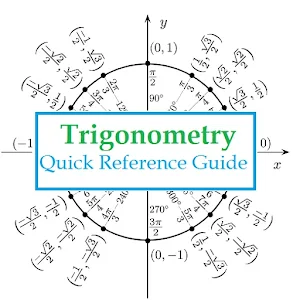# Trigonometry Quick Reference - Lumos Educational App Store4.21
Price -NA
\$A

#### DESCRIPTION:

Trigonometry Quick reference guide has all the important formulas and concepts covered to help the student review them on regular basis. Topics include: a) Definitions - Right Triangle Definition and Unit Triangle definition b) Facts and properties of trigonometric functions i.e., Domain, Range and period c) Formulas and Identities (includes Tangent and Cotangent Identities, Reciprocal Identities, Pythagorean Identities, Even/Odd Formulas, Periodic formulas, Double Angle formulas, Degree to radian formulas, Half-angle formulas, Sum and Difference formulas, Product to sum formulas, Sum to

#### OVERVIEW:

Trigonometry Quick Reference is a free educational mobile app By Nuzedd.It helps students in grades HS practice the following standards HSG.SRT.C.6,HSG.SRT.C.7,HSG.SRT.C.8.

This page not only allows students and teachers download Trigonometry Quick Reference but also find engaging Sample Questions, Videos, Pins, Worksheets, Books related to the following topics.

1. HSG.SRT.C.6 : Understand that by similarity, side ratios in right triangles are properties of the angles in the triangle, leading to definitions of trigonometric ratios for acute angles.

2. HSG.SRT.C.7 : Explain and use the relationship between the sine and cosine of complementary angles..

3. HSG.SRT.C.8 : Use trigonometric ratios and the Pythagorean Theorem to solve right triangles in applied problems.

HS

#### STANDARDS:

HSG.SRT.C.6
HSG.SRT.C.7
HSG.SRT.C.8
HSG.SRT.D.9
HSG.SRT.D.10
HSG.SRT.D.11
HSF.TF.A.1
HSF.TF.A.2
HSF.TF.A.3
HSF.TF.A.4
HSF.TF.B.5
HSF.TF.B.6
HSF.TF.B.7
HSF.TF.C.8
HSF.TF.C.9

Developer: Nuzedd

Software Version: 1.8

Category: Education

### RELATED APPSEdSearch WebSearch